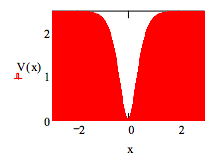Skip to main content

# 449: Variational Method for the Feshbach Potential

Define potential energy: V0 = 2.5 d = 0.5 $$V(x) = V_o \tanh \left( \dfrac{x}{d}\right)^2$$

Display potential energy:Choose Gaussian trial wavefunction:

$\psi (x, \beta ) = \left( \frac{2 \beta}{ \pi} \right) ^{ \frac{1}{4}} exp ( - \beta x^2)$

Demonstrate that the trial wavefunction is normalized.

$\int_{- \infty}^{ \infty} \psi (x, \beta )^2 dx~~~assume,~ \beta > 0 \rightarrow 1$

Evaluate the variational integral.

$E( \beta ) = \int_{ - \infty}^{ \infty} \psi (x, \beta ) \frac{-1}{2} \frac{d^2}{dx^2} \psi (x, \beta ) dx + \int_{- \infty}^{ \infty} V(x) \psi (x, \beta )^2 dx$

Minimize the energy integral with respect to the variational parameter, $$\beta$$.

$$\beta$$ = 1 $$\beta$$ = Minimize (E, $$\beta$$) $$\beta$$ = 0.913 E( $$\beta$$) = 1.484

Calculate the % error given that numerical integration of Schrödingerʹs equation (see next tutorial) yields E = 1.44949 Eh.

$\frac{E( \beta ) - 1.44949}{1.44949} \times 100 = 2.36$

Display wavefunction in the potential well.Calculate the probability that tunneling is occurring.

$V(x) = 1.484 |_{float,~3}^{solve,~x} \rightarrow {\begin{pmatrix} -1.511 \\ 0 .511 \end{pmatrix}}$

$2 \int_{0.511}^{ \infty} \psi (x, \beta )^2 dx = 0.329$

• Was this article helpful?